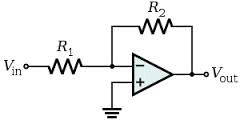## Op Amp Gain

Choose Inverting or Non-Inverting and enter two of the following: R1, R2 and K. Then click on "Get  x" for the desired parameter.

 SchematicConfig Non-Inverting Inverting

 R1 Ohms R2 Ohms K = Vout/Vin (V/V)

 Equations Non-Inverting Inverting K = R2/R1+1 R2 = R1*(K-1) R1 = R2/(K-1) K = -R2/R1 R1 = -R2*K R1 = -R2/K

To run or modify on your PC, download a simple version of this file with image (*.zip).
For a quick tour of some JavaScript / HTML basics, check out Inside the Code.

### JavaScript

This code features 3 separate function calls, one per "Get_x" button. Here's the code for the "Get R1" button.

```//////////////////////////////////////////////
// calc R1
//////////////////////////////////////////////
function getR1_OpAmpGain() {
// get values directly from form
var R2=document.myForm.R2.value;
var K=document.myForm.K.value;
var R1;

// calc non inverting
if (document.myForm.config.checked) {
if(K>0) R1=R2/(K-1);
else alert("K must be POSITIVE.") ;
}

// calc inverting
if (document.myForm.config.checked) {
if(K<0) R1=-R2/K;
else alert("K must be NEGATIVE.") ;
}
// place in text box
document.myForm.R1.value = (R1).toPrecision(4);
}
```

The two radio buttons, both named "config", create an array of elements config and config for the buttons named "Non-Inverting" and "Inverting". You can use the array to determine which button was checked as shown in the code above.

Some error checking was added to avoid non-sensical results. Basically, the gains are checked for proper polarity such that R1 and R2 are calculated correctly. Otherwise, an "alert" box pops up with a useful message.

### HTML

Here's the HTML for the radio butons to select the amplifier configuration. Note that both are named "config". You can set which button is initially selected by including "checked" in the input line.